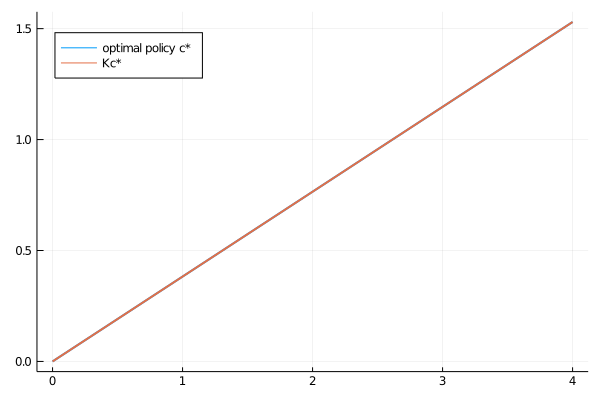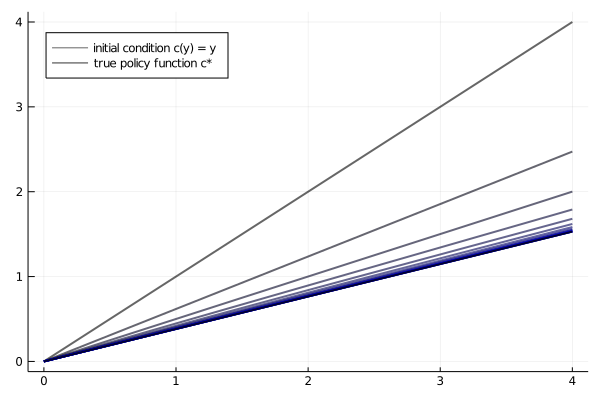# Optimal Growth III: The Endogenous Grid Method¶

## Overview¶

We solved the stochastic optimal growth model using

We found time iteration to be significantly more accurate at each step.

In this lecture we’ll look at an ingenious twist on the time iteration technique called the endogenous grid method (EGM).

EGM is a numerical method for implementing policy iteration invented by Chris Carroll.

It is a good example of how a clever algorithm can save a massive amount of computer time.

(Massive when we multiply saved CPU cycles on each implementation times the number of implementations worldwide)

The original reference is [Car06].

## Key Idea¶

Let’s start by reminding ourselves of the theory and then see how the numerics fit in.

### Theory¶

Take the model set out in the time iteration lecture, following the same terminology and notation.

The Euler equation is

$$(u'\circ c^*)(y) = \beta \int (u'\circ c^*)(f(y - c^*(y)) z) f'(y - c^*(y)) z \phi(dz) \tag{1}$$

As we saw, the Coleman operator is a nonlinear operator $K$ engineered so that $c^*$ is a fixed point of $K$.

It takes as its argument a continuous strictly increasing consumption policy $g \in \Sigma$.

It returns a new function $Kg$, where $(Kg)(y)$ is the $c \in (0, \infty)$ that solves

$$u'(c) = \beta \int (u' \circ g) (f(y - c) z ) f'(y - c) z \phi(dz) \tag{2}$$

### Exogenous Grid¶

As discussed in the lecture on time iteration, to implement the method on a computer we need numerical approximation.

In particular, we represent a policy function by a set of values on a finite grid.

The function itself is reconstructed from this representation when necessary, using interpolation or some other method.

Previously, to obtain a finite representation of an updated consumption policy we

• fixed a grid of income points $\{y_i\}$
• calculated the consumption value $c_i$ corresponding to each $y_i$ using (2) and a root finding routine

Each $c_i$ is then interpreted as the value of the function $K g$ at $y_i$.

Thus, with the points $\{y_i, c_i\}$ in hand, we can reconstruct $Kg$ via approximation.

Iteration then continues…

### Endogenous Grid¶

The method discussed above requires a root finding routine to find the $c_i$ corresponding to a given income value $y_i$.

Root finding is costly because it typically involves a significant number of function evaluations.

As pointed out by Carroll [Car06], we can avoid this if $y_i$ is chosen endogenously.

The only assumption required is that $u'$ is invertible on $(0, \infty)$.

The idea is this:

First we fix an exogenous grid $\{k_i\}$ for capital ($k = y - c$).

Then we obtain $c_i$ via

$$c_i = (u')^{-1} \left\{ \beta \int (u' \circ g) (f(k_i) z ) \, f'(k_i) \, z \, \phi(dz) \right\} \tag{3}$$

where $(u')^{-1}$ is the inverse function of $u'$.

Finally, for each $c_i$ we set $y_i = c_i + k_i$.

It is clear that each $(y_i, c_i)$ pair constructed in this manner satisfies (2).

With the points $\{y_i, c_i\}$ in hand, we can reconstruct $Kg$ via approximation as before.

The name EGM comes from the fact that the grid $\{y_i\}$ is determined endogenously.

## Implementation¶

Let’s implement this version of the Coleman operator and see how it performs.

### The Operator¶

Here’s an implementation of $K$ using EGM as described above.

### Setup¶

In :
using InstantiateFromURL
# optionally add arguments to force installation: instantiate = true, precompile = true
github_project("QuantEcon/quantecon-notebooks-julia", version = "0.8.0")

In :
using LinearAlgebra, Statistics
using BenchmarkTools, Interpolations, Parameters, Plots, QuantEcon, Random, Roots
gr(fmt = :png);

In :
function coleman_egm(g, k_grid, β, u′, u′_inv, f, f′, shocks)

# Allocate memory for value of consumption on endogenous grid points
c = similar(k_grid)

# Solve for updated consumption value
for (i, k) in enumerate(k_grid)
vals = u′.(g.(f(k) * shocks)) .* f′(k) .* shocks
c[i] = u′_inv(β * mean(vals))
end

# Determine endogenous grid
y = k_grid + c  # y_i = k_i + c_i

# Update policy function and return
Kg = LinearInterpolation(y,c, extrapolation_bc=Line())
Kg_f(x) = Kg(x)
return Kg_f
end

Out:
coleman_egm (generic function with 1 method)

Note the lack of any root finding algorithm.

We’ll also run our original implementation, which uses an exogenous grid and requires root finding, so we can perform some comparisons

In :
function K!(Kg, g, grid, β, u′, f, f′, shocks)

# This function requires the container of the output value as argument Kg

# Construct linear interpolation object #
g_func = LinearInterpolation(grid, g, extrapolation_bc = Line())

# solve for updated consumption value #
for (i, y) in enumerate(grid)
function h(c)
vals = u′.(g_func.(f(y - c) * shocks)) .* f′(y - c) .* shocks
return u′(c) - β * mean(vals)
end
Kg[i] = find_zero(h, (1e-10, y - 1e-10))
end
return Kg
end

# The following function does NOT require the container of the output value as argument
K(g, grid, β, u′, f, f′, shocks) =
K!(similar(g), g, grid, β, u′, f, f′, shocks)

Out:
K (generic function with 1 method)

Let’s test out the code above on some example parameterizations, after the following imports.

### Testing on the Log / Cobb–Douglas case¶

As we did for value function iteration and time iteration, let’s start by testing our method with the log-linear benchmark.

The first step is to bring in the model that we used in the Coleman policy function iteration

In :
# model

Model = @with_kw (α = 0.65, # productivity parameter
β = 0.95, # discount factor
γ = 1.0,  # risk aversion
μ = 0.0,  # lognorm(μ, σ)
s = 0.1,  # lognorm(μ, σ)
grid_min = 1e-6, # smallest grid point
grid_max = 4.0,  # largest grid point
grid_size = 200, # grid size
u = γ == 1 ? log : c->(c^(1-γ)-1)/(1-γ), # utility function
u′ = c-> c^(-γ), # u'
f = k-> k^α, # production function
f′ = k -> α*k^(α-1), # f'
grid = range(grid_min, grid_max, length = grid_size)) # grid

Out:
##NamedTuple_kw#253 (generic function with 2 methods)

Next we generate an instance

In :
mlog = Model(); # Log Linear model


We also need some shock draws for Monte Carlo integration

In :
Random.seed!(42); # For reproducible behavior.

shock_size = 250     # Number of shock draws in Monte Carlo integral
shocks = exp.(mlog.μ .+ mlog.s * randn(shock_size));


As a preliminary test, let’s see if $K c^* = c^*$, as implied by the theory

In :
c_star(y) = (1 - mlog.α * mlog.β) * y

# some useful constants
ab = mlog.α * mlog.β
c1 = log(1 - ab) / (1 - mlog.β)
c2 = (mlog.μ + mlog.α * log(ab)) / (1 - mlog.α)
c3 = 1 / (1 - mlog.β)
c4 = 1 / (1 - ab)

v_star(y) = c1 + c2 * (c3 - c4) + c4 * log(y)

Out:
v_star (generic function with 1 method)
In :
function verify_true_policy(m, shocks, c_star)
k_grid = m.grid
c_star_new = coleman_egm(c_star, k_grid, m.β, m.u′, m.u′, m.f, m.f′, shocks)

plt = plot()
plot!(plt, k_grid, c_star.(k_grid), lw = 2, label = "optimal policy c*")
plot!(plt, k_grid, c_star_new.(k_grid), lw = 2, label = "Kc*")
plot!(plt, legend = :topleft)
end

Out:
verify_true_policy (generic function with 1 method)
In :
verify_true_policy(mlog, shocks, c_star)

Out:Notice that we’re passing u′ to coleman_egm twice.

The reason is that, in the case of log utility, $u'(c) = (u')^{-1}(c) = 1/c$.

Hence u′ and u′_inv are the same.

We can’t really distinguish the two plots.

In fact it’s easy to see that the difference is essentially zero:

In :
c_star_new = coleman_egm(c_star, mlog.grid, mlog.β, mlog.u′,
mlog.u′, mlog.f, mlog.f′, shocks)
maximum(abs(c_star_new(g) - c_star(g)) for g in mlog.grid)

Out:
1.3322676295501878e-15

Next let’s try iterating from an arbitrary initial condition and see if we converge towards $c^*$.

Let’s start from the consumption policy that eats the whole pie: $c(y) = y$

In :
n = 15
function check_convergence(m, shocks, c_star, g_init, n_iter)
k_grid = m.grid
g = g_init
plt = plot()
plot!(plt, m.grid, g.(m.grid),
color = RGBA(0,0,0,1), lw = 2, alpha = 0.6, label = "initial condition c(y) = y")
for i in 1:n_iter
new_g = coleman_egm(g, k_grid, m.β, m.u′, m.u′, m.f, m.f′, shocks)
g = new_g
plot!(plt, k_grid, new_g.(k_grid), alpha = 0.6, color = RGBA(0,0,(i / n_iter), 1),
lw = 2, label = "")
end

plot!(plt, k_grid, c_star.(k_grid),
color = :black, lw = 2, alpha = 0.8, label = "true policy function c*")
plot!(plt, legend = :topleft)
end

Out:
check_convergence (generic function with 1 method)
In :
check_convergence(mlog, shocks, c_star, identity, n)

Out:We see that the policy has converged nicely, in only a few steps.

## Speed¶

Now let’s compare the clock times per iteration for the standard Coleman operator (with exogenous grid) and the EGM version.

We’ll do so using the CRRA model adopted in the exercises of the Euler equation time iteration lecture.

Here’s the model and some convenient functions

In :
mcrra = Model(α = 0.65, β = 0.95, γ = 1.5)
u′_inv(c) = c^(-1 / mcrra.γ)

Out:
u′_inv (generic function with 1 method)

Here’s the result

In :
crra_coleman(g, m, shocks) = K(g, m.grid, m.β, m.u′, m.f, m.f′, shocks)
crra_coleman_egm(g, m, shocks) = coleman_egm(g, m.grid, m.β, m.u′,
u′_inv, m.f, m.f′, shocks)
function coleman(m = m, shocks = shocks; sim_length = 20)
g = m.grid
for i in 1:sim_length
g = crra_coleman(g, m, shocks)
end
return g
end
function egm(m, g = identity, shocks = shocks; sim_length = 20)
for i in 1:sim_length
g = crra_coleman_egm(g, m, shocks)
end
return g.(m.grid)
end

Out:
egm (generic function with 3 methods)
In :
@benchmark coleman($mcrra)  Out: BenchmarkTools.Trial: memory estimate: 1.03 GiB allocs estimate: 615012 -------------- minimum time: 7.132 s (1.56% GC) median time: 7.132 s (1.56% GC) mean time: 7.132 s (1.56% GC) maximum time: 7.132 s (1.56% GC) -------------- samples: 1 evals/sample: 1 In : @benchmark egm($mcrra)

Out:
BenchmarkTools.Trial:
memory estimate:  18.50 MiB
allocs estimate:  76226
--------------
minimum time:     152.147 ms (0.00% GC)
median time:      157.500 ms (0.00% GC)
mean time:        158.227 ms (1.34% GC)
maximum time:     167.435 ms (3.40% GC)
--------------
samples:          32
evals/sample:     1

We see that the EGM version is about 30 times faster.

At the same time, the absence of numerical root finding means that it is typically more accurate at each step as well.